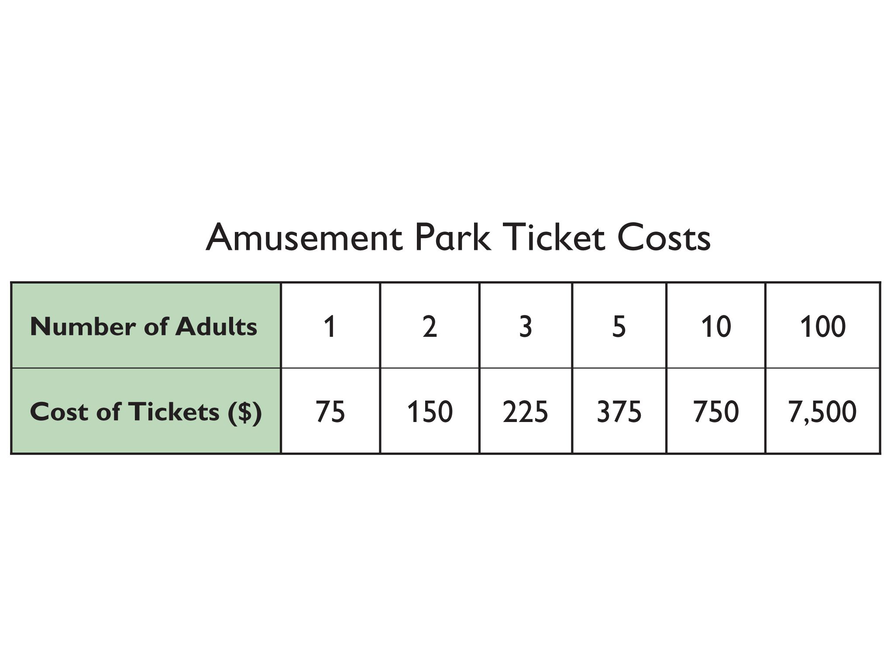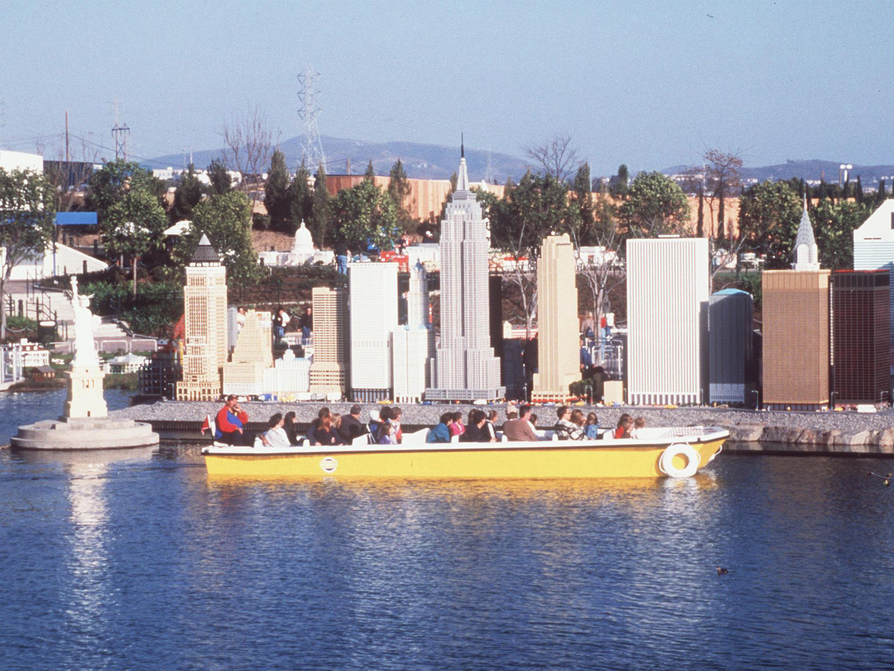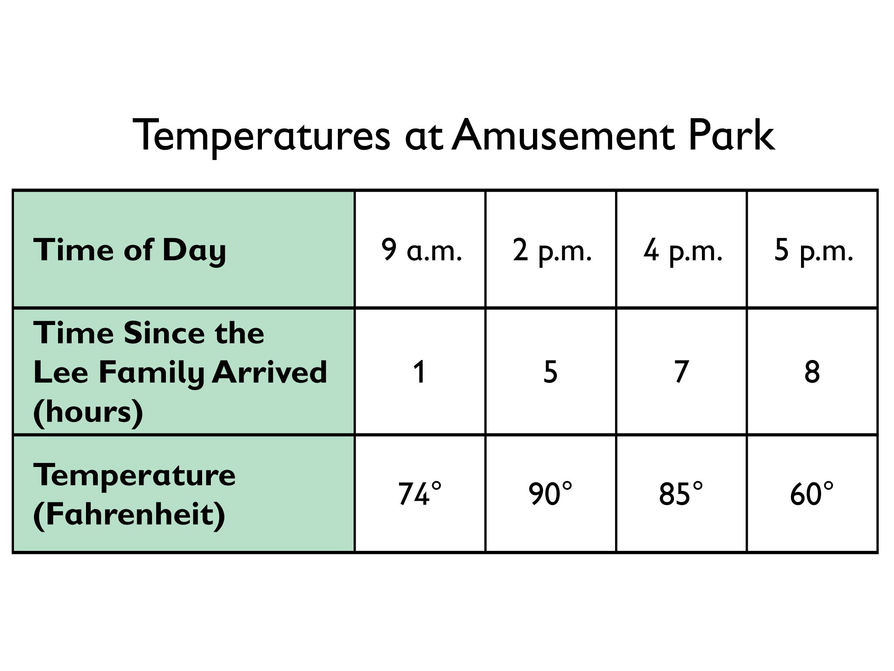Subject:
Ratios and Proportions
Material Type:
Lesson Plan
Level:
Middle School
7
Provider:
Pearson
Tags:
• Decimals
• Dimensions
• Distance
• Time
Language:
English
Media Formats:
Text/HTML

# Identifying Proportional Relationships## Overview

Students determine whether a relationship between two quantities that vary is a proportional relationship in three different situations: the relationship between the dimensions of the actual Empire State Building and a miniature model of the building; the relationship between the distance and time to travel to an amusement park; and the relationship between time and temperature at an amusement park.

# Key Concepts

When the ratio between two varying quantities remains constant, the relationship between the two quantities is called a proportional relationship. For a ratio A:B, the proportional relationship can be described as the collection of ratios equivalent to A:B, or cA:cB, where c is positive.

# Goals and Learning Objectives

• Identify proportional relationships.
• Explain why a situation represents a proportional relationship or why it does not.
• Determine missing values in a table of quantities based on a proportional relationship.

# Lesson Guide

• Introduce the situation and read the definition of a proportional relationship.
• Facilitate a class discussion about the values shown in the Amusement Park Ticket Costs table.

ELL: Allow and encourage the use of dictionaries or translation sites should students (especially ELLs) need them to better understand the topic and to improve their use of academic vocabulary.

# Mathematics

Connecting the definition of a proportional relationship to a set of real-world quantities is the mathematical focus of the start of the lesson: when the ratio between two varying quantities remains constant, the relationship between these two quantities is called a proportional relationship. The ratio that remains constant is the ratio between the number of tickets and the total cost of the tickets. This ratio is the ticket price: $75. Ask your students the following: • What is constant in this situation? (Answer: the ticket price) • What varies in this situation? (Answer: the number of adults and the cost of the tickets) • What quantities does the table show? (Answer: the number of adults and the cost of the tickets) ELL: In asking prompting questions, be sure to use adequate pace with ELLs, and be sure that they understand the meaning of the questions. ## Opening # A Park Ticket Discuss the following with your classmates. • At an amusement park in California, the cost of admission is$75.
• This table shows the costs of tickets for different numbers of adults.
• You can see from the table that the cost of the tickets is always 75 times the number of adults. You can express this relationship using the ratio 75:1.
• When the ratio between two varying quantities remains constant, the relationship between the two quantities is called a proportional relationship.
• The relationship between the number of adults and the cost of tickets is constant; thus, the relationship is a proportional relationship.# Lesson Guide

Discuss the Math Mission. Students will determine whether the relationship between two quantities that vary is a proportional relationship.

## Opening

Determine whether the relationship between two quantities that vary is a proportional relationship.

# Lesson Guide

Have students work in pairs on all problems and the presentation.

SWD: Make sure students analyze this information in the form of a table. Seeing the data clearly outlined will help students to better understand the concept. Also, they will be able to observe whether the ratios are in proportion.

# Interventions

Student attempts calculations, but is unsure what to multiply by what or what to divide by what.

• Think about the relationship between the two quantities. Which quantity is always going to have lesser values than the other quantity?
• Imagine that you are building a model car with a scale of 1:100. Would you multiply by 1,100 (or divide by 100) to identify the dimensions of your model?

• Yes, the table represents a proportional relationship because the ratio between each dimension of the real building, and the corresponding dimension of the miniature building is constant.

# Height of the Miniature

An amusement park has a miniature scale model of New York City. The dimensions of the buildings in the miniature city are proportional to the dimensions of the real buildings—that is, the ratio between the dimensions of the miniature buildings and the dimensions of the real buildings is constant.

The height of the real Empire State Building is 1,454 feet. Suppose that the miniature Empire State Building had been constructed using a 20:1 ratio or scale.

• Fill in the missing dimensions in the table based on this scale.
• Does the table represent a proportional relationship? Explain why or why not.INTERACTIVE: Using Scale to Determine Dimension

## Hint:

To find the height of the Empire State Building miniature, write an equation that sets the ratio 20 : 1 equal to the ratio of the height of the real building to the height of the miniature building.

# Lesson Guide

Have students work in pairs on all problems and the presentation.

# Interventions

In the driving to the amusement park problem, student treats 0.5 hours, in the first column of the table, as the unit rate.

• Look up a definition of unit rate.
• Which column in the table gives you Mr. Lee’s speed as it would normally be reported, in miles per hour?

• Yes, the table represents a proportional relationship because the ratio between distance traveled and time is constant.

# Driving to the Park

This table shows that Mr. Lee drove 72.5 miles in 1.5 hours on his way to the amusement park.

• Fill in the missing values in the table, assuming that Mr. Lee drove at a constant speed.
• Does the table represent a proportional relationship? Explain why or why not.

INTERACTIVE: Driving to the Park

## Hint:

What distance did Mr. Lee travel in 1 hour? How can you find out?

# Lesson Guide

Have students work in pairs on all problems and the presentation.

# Interventions

In the temperatures at the amusement park problem, student believes the temperature can be calculated from the given information in the table.

• Is the ratio between time and temperature the same in each column of the table? If not, it means there is not a constant ratio between the quantities in this situation.
• What patterns can you find in the values given in the table?
• When you look up a weather prediction, does it tell you what the actual temperature is going to be or a prediction based on available evidence?

• No, I can’t tell from the table what the temperature was at noon; I can guess it was probably around 80°F or 85°F.
• No, the table does not represent a proportional relationship because there is not a constant ratio between time and temperature (line is not straight) and the graph doesn’t pass through the origin.

# Temperature at the Park

The temperature went up and down on the day the Lee family went to the park. They arrived at the park at 8:00 in the morning.

• Can you use the information in the table to determine what the temperature was at 12 noon?
• Does the table represent a proportional relationship? Explain why or why not.## Hint:

Calculate each ratio in the table. Are they the same?

# Preparing for Ways of Thinking

Listen and look for students who:

• Use a range of approaches when finding the missing values in the tables, including approaches that involve apparently independent or disconnected calculations for each missing value, record keeping that documents a repeated calculation procedure, a clear understanding of what is proportional to what in each case, and the setting up of a formula that works in every case.
• Discuss what the constant ratio is in the first two problems.
• Discuss whether or not there is a constant ratio in the problem about the temperatures at the amusement park.

# Challenge Problem

• Answers will vary. Possible answer: The apparent size of an object depends on its distance from a viewer. When looking up at a tall building, the distance of the upper portions of the building makes the portions appear smaller than they are. So creating models of buildings with relatively smaller and smaller portion sizes as your eye moves up from the ground to the top creates a perception of greater height. Explanations may also include the fact that visitors to the amusement park include many children, whose height facilitates this illusion.

# Prepare a Presentation

Define proportional relationship in your own words. Use examples from the Work Time problems to support your definition.

# Challenge Problem

In the park, most of the buildings in the miniature of New York City were constructed using a scale of 1:20 or 1:40. But the builders of the miniature city decided that the miniature of the Empire State Building would be too tall if they used either of these scales. If they used a scale of 1:20, the miniature would be over 70 feet high; if they used a scale of 1:40, the miniature would be over 30 feet high.

The builders decided to construct the miniature of the Empire State Building using four different scales! They used a scale of 1:20 for the ground floors in order to “match” the size of the people on the street. They built the main body of the building using a scale of 1:40. Closer to the top, they used a scale of 1:60, and for the very top tower they used a scale of 1:80. With this combination of scales, the miniature Empire State Building stands at 20 feet tall.

• Why do you think the builders used different scales for different parts of the building? Explain your thinking.

# Lesson Guide

Ask for presentations from students who used a range of approaches to find the missing values in the tables, including those who kept organized records to document a repeated calculation procedure, those who set up a formula (or took initial steps towards setting up a formula), and those who demonstrated a clear understanding of what is proportional to what. Focus attention on the constant of proportionality in each of the situations by asking the following:

• What is proportional to what?
• What is the ratio (its value) that defines this relationship? (Tell students this value is called the constant of proportionality.)
• What is the meaning of the constant of proportionality?

There is no need to call attention to the fact that there are two constant values in each situation, $\frac{1}{20}$ and $\frac{20}{1}$ in the first problem and $\frac{48.33}{1}$ and $\frac{1}{48.33}$ in the second problem, but if students raise the issue, do affirm their thinking.

Then focus the discussion on the temperatures at the amusement park problem by asking for different ways of thinking about the situation, including any students who found each ratio of time to temperature. Discuss what is different in this situation by asking the following:

• How is temperature different from the quantities in the situations in the first two problems?
• How is the situation in this problem different mathematically from the situations in the first two problems?

SWD: During the student presentations, provide some students with a note-taking organizer that includes a space for:

• The presenters’ name
• The presenters’ answer to the problem
• The presenters’ explanation of the mathematics
• The student’s summary of the presentation in his or her own words
• Reflection on what was presented

This will help students when they reflect on the presentations.

# Ways of Thinking: Make Connections

Take notes about your classmates’ explanations of the relationships between the quantities in the problems.

## Hint:

• What are the quantities in this situation?
• What are the ratios that represent the quantities? Are the ratios equal?
• What does it mean when the ratios are equal?
• Do you see any patterns in the table? If so, describe them.

# A Possible Summary

Two quantities have a proportional relationship to one another if the ratios between corresponding values of the quantities all have the same value. This ratio is called a constant of proportionality.

One additional thing you might want to discuss is the following:

• What makes a relationship a proportional relationship?

SWD: Check to make sure that all students have this information in their notes. It may be helpful to provide some students with certain things to listen for during this portion of instruction.

Some students may need copies of the notes from this portion of the lesson.

# Summary of the Math: Proportional Relationships

Write a summary about proportional relationships.

## Hint:

• Do you explain what makes a relationship a proportional relationship?
• Do you explain how you can identify a proportional relationship in a table?
• Do you use the words ratio andconstant ?

# Lesson Guide

Have each student write a brief reflection before the end of the class. Review the reflections to find out how students determine whether two quantities that vary are proportional.

ELL: When writing the reflection, allow some additional time for ELLs to discuss with a partner before writing, so as to help them organize their thoughts. Allow ELLs who share the same native language to discuss in that language if they wish and to use a dictionary.

# Reflection

Write a reflection about the ideas discussed in class today. Use the sentence starter below if you find it to be helpful.

One way that I can figure out whether two quantities that vary are proportional is …